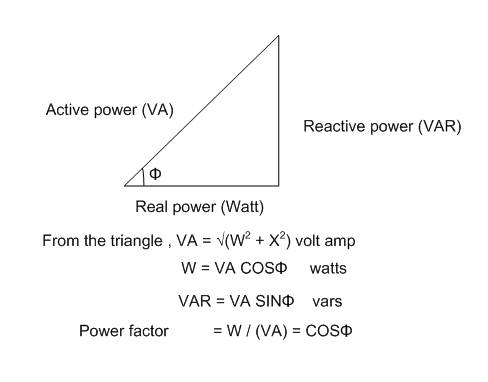# Power Factor

## Homework Statement

I understand power factor is defined as (average power)/(apparent power) or cos(theta v - theta i) where theta v = load voltage and theta i = load current. Also the power factor angle is equal to the load impedance.

I don't understand how the 2 definitions of power factor equal each other or why the power factor angle equals the load impedance.

I know it's a tall order but I would appreciate it if somebody could answer these questions. Alternatively a good website or book that explains this fully.

Your first statement means PF = Cos of the angle between V (the supply voltage) and the current vectors.
It does not equal the impedance but the angle between the impedance and the resistance vectors is the same as in my first sentence.
Hope this helps you to picture the vectors and the angle

See if this helpsThis is what I was after.

#### Attachments

• Pwr Factor Angle.pdf
61.7 KB · Views: 201